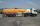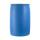# Square root + cylinder - math problems

#### Number of problems found: 9

• CylinderThe cylinder surface is 922 dm2, its height is equal to the radius of the base. Calculate height of this cylinder.
• Diameter = heightThe surface of the cylinder, the height of which is equal to the diameter of the base, is 4239 cm square. Calculate the cylinder volume.
• HoleIn the center of the cube with edge 14 cm we will drill cylinder shape hole. Volume of the hole must be 27% of the cube. What drill diameter should be chosen?
• Tank diameterA cylindrical tank has a volume of 60 hectoliters and is 2.5 meters deep. Calculate the tank diameter.
• Gasoline tank cylindricalWhat is the inner diameter of the tank, which is 8 m long and contains 40 cubic cubic meters of gasoline?
• Gold wireFrom one gram of gold was pulled wire 2.1 km length. What is it diameter if density of Au is ρ=19.5 g/cm3?
• Secret treasureScouts have a tent in the shape of a regular quadrilateral pyramid with a side of the base 4 m and a height of 3 m. Determine the radius r (and height h) of the container so that they can hide the largest possible treasure.
• Brass tubeThe outer perimeter of brass tube (ρ = 8.5 g/cm3) is 38 cm. Its mass is 5 kg, length 54 cm. What is the pipe wall thickness?
• TankIn the middle of a cylindrical tank with a bottom diameter 251 cm is standing rod which is 13 cm above the water surface. If we bank rod its end reach surface of the water just by the tank wall. How deep is the tank?

We apologize, but in this category are not a lot of examples.
Do you have an interesting mathematical word problem that you can't solve it? Submit a math problem, and we can try to solve it.

We will send a solution to your e-mail address. Solved examples are also published here. Please enter the e-mail correctly and check whether you don't have a full mailbox.

Please do not submit problems from current active competitions such as Mathematical Olympiad, correspondence seminars etc...

Square root - math word problems. Cylinder Problems.